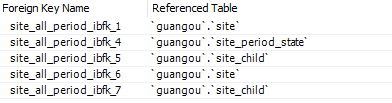# Django ORM 入门笔记

Django 发布于 Mar 9, 2021 更新于 Jul 19, 2022

## ORM 基础概念

### 简单了解 ORM

Object Relational Mapping 对象关系映射，简称ORM。

ORM通过使用描述对象和数据库之间映射的元数据，将程序中的对象自动持久化到关系数据库中。ORM在业务逻辑层和数据库层之间充当了桥梁的作用。

### ORM 对象和数据库结构的对应关系

``````class SiteAllPeriod(models.Model):
periodid = models.AutoField(db_column='periodID', primary_key=True)
week = models.IntegerField()
siteperiodid = models.IntegerField(db_column='sitePeriodID')
siteid = models.ForeignKey(Site, models.DO_NOTHING, db_column='siteID', related_name='%(class)s_siteid')
sitename = models.ForeignKey(Site, models.DO_NOTHING, db_column='siteName', related_name='%(class)s_sitename')
date = models.DateField()
childsiteid = models.ForeignKey('SiteChild', models.DO_NOTHING, db_column='childSiteID', blank=True, null=True, related_name='%(class)s_childsiteid')
childsitename = models.ForeignKey('SiteChild', models.DO_NOTHING, db_column='childSiteName', blank=True, null=True, related_name='%(class)s_childsitename')
starttime = models.TimeField(db_column='startTime', blank=True, null=True)
endtime = models.TimeField(db_column='endTime', blank=True, null=True)
stateid = models.ForeignKey('SitePeriodState', models.DO_NOTHING, db_column='stateID', blank=True, null=True, related_name='%(class)s_stateid')
prompt = models.CharField(max_length=50, blank=True, null=True)
price = models.DecimalField(max_digits=10, decimal_places=2, blank=True, null=True)
studentprice = models.DecimalField(db_column='studentPrice', max_digits=10, decimal_places=2, blank=True, null=True)
staffprice = models.DecimalField(db_column='staffPrice', max_digits=10, decimal_places=2, blank=True, null=True)lowercase.
orderuserpaymethod = models.CharField(db_column='orderUserPayMethod', max_length=30, blank=True, null=True)
needsubcard = models.IntegerField(db_column='needSubcard', blank=True, null=True)
teachingperiodname = models.CharField(db_column='teachingPeriodName', max_length=30, blank=True, null=True)
teacher = models.CharField(max_length=30, blank=True, null=True)

class Meta:
managed = False
db_table = 'site_all_period'
``````• 类对应某个特定的表
• 类的实例对应着一条记录
• 类的属性对应着表内的字段
• 类属性的类型对应着表内字段的类型
• 类属性类型构造时的参数与字段的参数一一对应
• 外键拥有特殊的类型
• 外键类型直接指向另一个 ORM 模型（如果另一个模型在本模型下方，需要用单引号包裹）

【知识链接】👇

Django 官方文档 – ORM 中的模型

Django 官方文档 – ORM 中的字段

## 在 Django 中配置 ORM 模型

### 创建一个 ORM 模型

``````from django.db import models

class Person(models.Model):
first_name = models.CharField(max_length=30)
last_name = models.CharField(max_length=30)
``````

`first_name``last_name` 是模型的 字段。每个字段都被指定为一个类属性，并且每个属性映射为一个数据库列。

``````CREATE TABLE myapp_person (
"id" serial NOT NULL PRIMARY KEY,
"first_name" varchar(30) NOT NULL,
"last_name" varchar(30) NOT NULL
);
``````

### 使用新创建的模型

``````INSTALLED_APPS = [
#...
'myapp',
#...
]
``````

### 使用 Meta 信息指定命名表

``````from django.db import models

class Person(models.Model):
first_name = models.CharField(max_length=30)
last_name = models.CharField(max_length=30)

class Meta:
db_table = 'person'
``````

### 使用已有数据库自动建立 ORM 模型

• Django ORM 的另一强大之处在于它可以双向构造映射
• 创建了模型，可以生成表；同样的，设计了表，可以生成模型

``````python manage.py dbshell
``````

``````python manage.py inspectdb > models.py
``````

## 在 Django 中使用 ORM 完成数据库操作

``````class SiteAllPeriod(models.Model):
periodid = models.AutoField(db_column='periodID', primary_key=True)
week = models.IntegerField()
siteperiodid = models.IntegerField(db_column='sitePeriodID')
siteid = models.ForeignKey(Site, models.DO_NOTHING, db_column='siteID', related_name='%(class)s_siteid')
sitename = models.ForeignKey(Site, models.DO_NOTHING, db_column='siteName', related_name='%(class)s_sitename')
date = models.DateField()
childsiteid = models.ForeignKey('SiteChild', models.DO_NOTHING, db_column='childSiteID', blank=True, null=True, related_name='%(class)s_childsiteid')
childsitename = models.ForeignKey('SiteChild', models.DO_NOTHING, db_column='childSiteName', blank=True, null=True, related_name='%(class)s_childsitename')
starttime = models.TimeField(db_column='startTime', blank=True, null=True)
endtime = models.TimeField(db_column='endTime', blank=True, null=True)
stateid = models.ForeignKey('SitePeriodState', models.DO_NOTHING, db_column='stateID', blank=True, null=True, related_name='%(class)s_stateid')
prompt = models.CharField(max_length=50, blank=True, null=True)
price = models.DecimalField(max_digits=10, decimal_places=2, blank=True, null=True)
studentprice = models.DecimalField(db_column='studentPrice', max_digits=10, decimal_places=2, blank=True, null=True)
staffprice = models.DecimalField(db_column='staffPrice', max_digits=10, decimal_places=2, blank=True, null=True)lowercase.
orderuserpaymethod = models.CharField(db_column='orderUserPayMethod', max_length=30, blank=True, null=True)
needsubcard = models.IntegerField(db_column='needSubcard', blank=True, null=True)
teachingperiodname = models.CharField(db_column='teachingPeriodName', max_length=30, blank=True, null=True)
teacher = models.CharField(max_length=30, blank=True, null=True)

class Meta:
managed = False
db_table = 'site_all_period'
``````

### 1. SELECT * FROM site_all_period

ORM中，使用以下语句获取记录

``````querySet = SiteAllPeriod.objects.all()
``````

SQL 语句查询返回的是一个记录列表，而 ORM 查询的返回值是`QuerySet`，顾名思义，这是一个储存查询结果的集合。

#### 了解 `QuerySet`

`QuerySet`和 Python 标准的 list 类型有些相似，使用时很多时候可以把它当作一个 list 来处理，比如可以使用`for ... in ...`遍历选择到的记录，或者使用下标访问`index`位置的记录（如果记录有序，这个的用处就比较大了）。

• 仅仅创建`QuerySet`不会触发数据库操作，只有在`QuerySet`被使用（读取具体内容）时，才会执行对应的 SQL 语句。

• `QuerySet`是可以迭代的，比如你可以使用`records = SiteAllPeriod.objects.all().all().all().all().all()`（逃）

请牢记它可以被迭代，它的迭代特性可以用来组装一个复杂的 SQL 语句。看文档应该是会合并成单 SQL 语句，有待验证，好奇可以QQ联络我让我验证。

• `QuerySet`具有缓存功能，如果事先创建了`QuerySet`对象，那么对它进行遍历不会反复进行数据库操作，而是会对已有的`QuerySet`对象进行遍历。

具体缓存机制请参阅 Django 官方文档 – ORM QuerySet 缓存机制

### 2. SELECT * FROM site_all_period WHERE week=1

ORM中，使用以下语句获取记录

``````querySet = SiteAllPeriod.objects.filter(week=1) # 使用参数名确定对象属性(字段)
``````

#### TIP

``````querySet = SiteAllPeriod.objects.filter(siteid=1)
``````

``````querySet = SiteAllPeriod.objects.filter(siteid_id=1) # 外键属性+_id才能获取到字段的值
``````

### 3. SELECT JOIN 连接查询/跨表查询

``````querySet = SiteAllPeriod.objects.filter(siteid__courtid_id=1)
``````

### 4. 模型对象之间的访问

Django 的 ORM 模型还有个不错的地方就是：

``````siteAllPeriod_record = SiteAllPeriod.objects.filter(siteid_id=1).first() # 选择 siteid = 1 的记录，并拿取第一个（当然这种情况也只有一个）
courtid_of_this_period = siteAllPeriod_record.siteid.courtid_id
"""
siteid 是外键属性，访问该属性会直接返回对应的对象

"""
``````

### 6. 记录的删除

5一模一样，就是把`save()`换成`delete()`